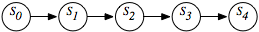### 6.5.1 Markov Chains

A Markov chain is a special sort of belief network used to represent sequences of values, such as the sequence of states in a dynamic system or the sequence of words in a sentence.Figure 6.13: A Markov chain as a belief network

Figure 6.13 shows a generic Markov chain as a belief network. The network does not have to stop at stage s4, but it can be extended indefinitely. The belief network conveys the independence assumption

P(St+1|S0,...,St)=P(St+1|St),

which is called the Markov assumption.

Often, St represents the state at time t. Intuitively, St conveys all of the information about the history that can affect the future states. At St, you can see that "the future is conditionally independent of the past given the present."

A Markov chain is stationary if the transition probabilities are the same for each time point [i.e., for all t>0, t'>0, P(St+1|St)=P(St'+1|St')]. To specify a stationary Markov chain, two conditional probabilities must be specified:

• P(S0) specifies initial conditions.
• P(St+1|St) specifies the dynamics, which is the same for each t ≥ 0.

Stationary Markov chains are of interest because

• They provide a simple model that is easy to specify.
• The assumption of stationarity is often the natural model, because the dynamics of the world typically does not change in time. If the dynamics does change in time, it is usually because some other feature exists that could also be modeled.
• The network can extend indefinitely. Specifying a small number of parameters can give an infinite network. You can ask queries or make observations about any arbitrary points in the future or the past.

To determine the probability distribution of state Si, VE can be used to sum out the preceding variables. Note that the variables after Si are irrelevant to the probability of Si and need not be considered. This computation is normally specified as matrix multiplication, but note that matrix multiplication is a simple form of VE. Similarly, to compute P(Si|Sk), where k>i, only the variables before Sk need to be considered.